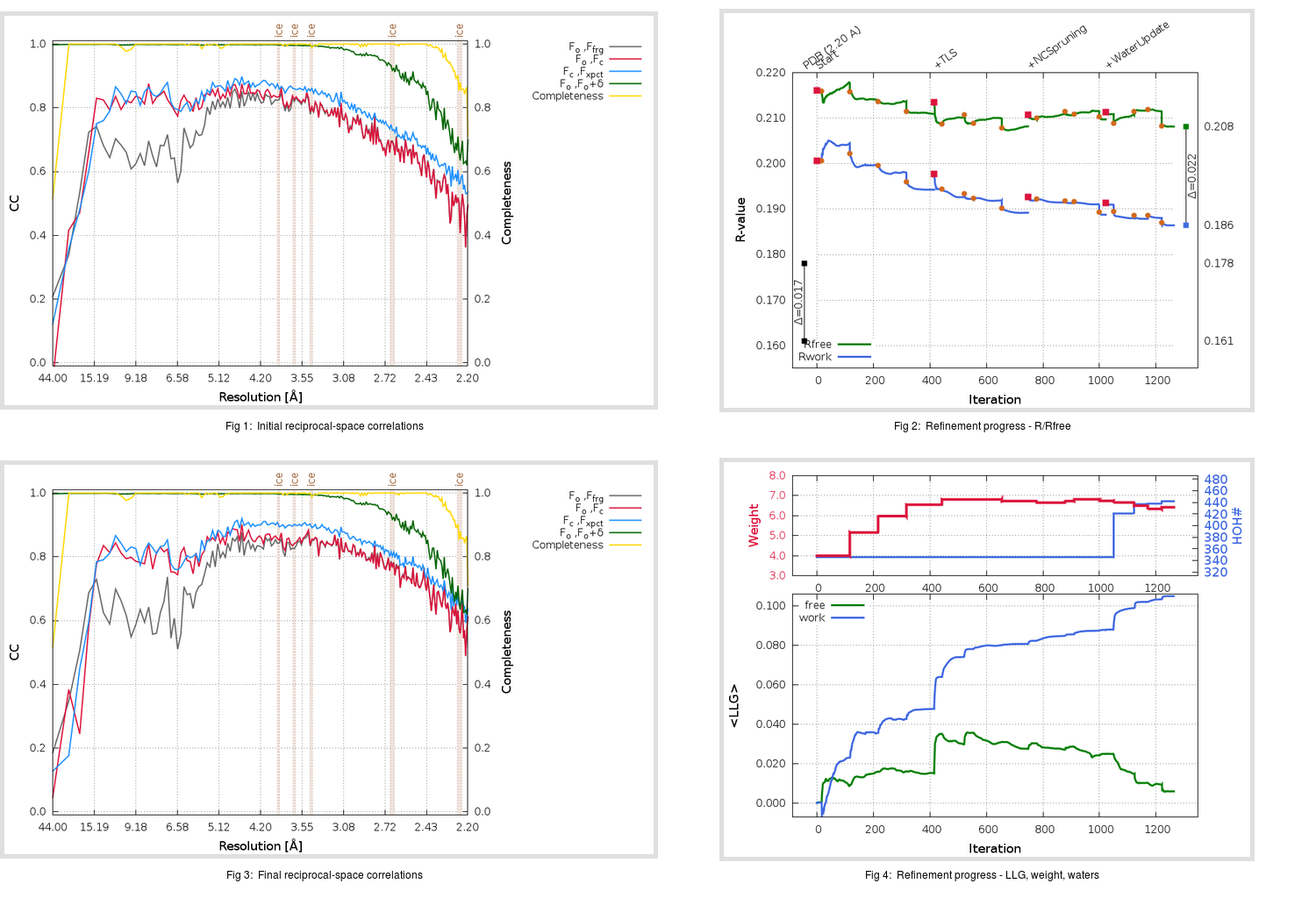Content:

```    Diffraction limits & principal axes of ellipsoid fitted to diffraction cut-off surface:
2.516         1.0000   0.0000   0.0000       0.894 a* - 0.447 b*
2.516         0.0000   1.0000   0.0000       b*
2.629         0.0000   0.0000   1.0000       c*
```

## Deposited

` `
 Date deposited Date data collection Resolution R, Rfree 20200220 20200219 2.20 0.1580 0.1780

Molprobity (CCP4 7.0 version) summary:

```Ramachandran outliers =   0.00 %
favored =  97.83 %
Rotamer outliers      =   1.43 %
C-beta deviations     =     0
Clashscore            =   1.76
RMS(bonds)            =   0.0036
RMS(angles)           =   0.91
MolProbity score      =   1.09
Resolution            =   2.20
R-work                =   0.1580
R-free                =   0.1780
```

```Number of waters      =   346

<B> (all atoms) =   43.98 ( sd =   15.88 ) for       6009 non-hydrogen atoms
<B>   (protein) =   43.66 ( sd =   15.94 ) for       5589 non-hydrogen atoms
<B>     (water) =   43.95 ( sd =   10.49 ) for        346 non-hydrogen atoms
<B>    (others) =   70.00 ( sd =   15.95 ) for         74 non-hydrogen atoms

B min/max       (all non-hydrogen atoms) =   18.60 /  118.06
B min/max   (protein non-hydrogen atoms) =   18.60 /  118.06
B min/max     (water non-hydrogen atoms) =   20.64 /   77.41
B min/max     (other non-hydrogen atoms) =   49.72 /   94.87
```

## BUSTER (re-)refinement

` `

Molprobity (CCP4 7.0 version) summary:

```Ramachandran outliers =   0.00 %
favored =  98.41 %
Rotamer outliers      =   1.91 %
C-beta deviations     =     1
Clashscore            =   2.56
RMS(bonds)            =   0.0127
RMS(angles)           =   1.56
MolProbity score      =   1.25
Resolution            =   2.20
R-work                =   0.1867
R-free                =   0.2085
```

```Number of waters      =   442

<B> (all atoms) =   44.95 ( sd =   13.22 ) for       6105 non-hydrogen atoms
<B>   (protein) =   44.23 ( sd =   12.78 ) for       5589 non-hydrogen atoms
<B>     (water) =   49.90 ( sd =   13.28 ) for        442 non-hydrogen atoms
<B>    (others) =   71.43 ( sd =   17.31 ) for         74 non-hydrogen atoms

B min/max       (all non-hydrogen atoms) =   19.46 /   99.10
B min/max   (protein non-hydrogen atoms) =   23.05 /   92.81
B min/max     (water non-hydrogen atoms) =   19.46 /   92.89
B min/max     (other non-hydrogen atoms) =   53.18 /   99.10
```

Refinement progression:Results:

` `
 File Remark 6VWW_aB_refine.01_04_refine.pdb.gz exact refinement commands are in header 6VWW_aB_refine.01_04_refine.mtz.gz including original deposited data and several re-refinement map coefficients 6VWW_aB_refine.01_04_BUSTER_model.cif.gz including any non-standard compound restraints 6VWW_aB_refine.01_04_BUSTER_refln.cif.gz